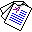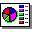# Generality Relations in Answer Set Programming

## Katsumi Inoue and Chiaki Sakama

Proceedings of the 22nd International Conference on Logic Programming (ICLP'06), Lecture Notes in Computer Science 4079, Springer-Verlag, pages 211-225, 2006.

## Abstract

This paper studies generality relations on logic programs. Intuitively, a program P1 is more general than another program P2 if P1 gives us more information than P2. In this paper, we define various kinds of generality relations over nonmonotonic programs in the context of answer set programming. The semantic properties of generality relations are investigated based on domain theory, and both a minimal upper bound and a maximal lower bound are constructed for two logic programs. We also introduce the concept of strong generality between logic programs and investigate its relationships to strong equivalence. These results provide a basic theory to compare the degree of incompleteness between nonmonotonic logic programs, and also have important applications to inductive logic programming and multi-agent systems.Full Paper (PDF 232K)Slide (pdf 215K) , © Springer-Verlag